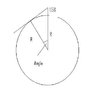# Laying on the beach while sunseting question

djkinney

## Homework Statement

Suppose that, while lying on a beach near the equator of a far-off planet watching the sun set over a calm ocean, you start a stopwatch just as the top of the sun disappears. You then stand, elevating your eyes by a height H = 1.58 m, and stop the watch when the top of the sun again disappears. If the elapsed time is t = 10.9 s, what is the radius r of the planet to two significant figures? Notice that duration of a solar day at the far-off planet is the same that is on Earth.

86400 s=1 day

## The Attempt at a Solution

I attempted to solve this by setting the angle, but I think I got the wrong angle. (.0454°)
cos(0.0454°)=((r)/(r+h)) h=1.58

Homework Helper
Hi djkinney,

how did you get the angle?

ehild

djkinney
Hi djkinney,

how did you get the angle?

ehild

Hey,
Well I'm not quite sure as to how to get the angle. I tried two different ways so far: First, I tried: 10.9s/86400s=1.26e-4°. The second was me trying something crazy and doesn't make any sense: 10.9s*(1min/60s)*(1hr/60min)*(360/24hr)=.0454°

Homework Helper
Hey,
Well I'm not quite sure as to how to get the angle. I tried two different ways so far: First, I tried: 10.9s/86400s=1.26e-4°. The second was me trying something crazy and doesn't make any sense: 10.9s*(1min/60s)*(1hr/60min)*(360/24hr)=.0454°

The first method will be all right, but it is not degrees. 1.26e-4 is the ratio to the total revolution, which corresponds to 360 degrees.

ehild

djkinney
The first method will be all right, but it is not degrees. 1.26e-4 is the ratio to the total revolution, which corresponds to 360 degrees.

ehild

Okay, so that means that 1.26E^-4*360°=.04536° for the angle? Then I would still be getting my final answer as 1.5E^3

Homework Helper
Well, what is that angle and how is it related to the radius of the planet and your height?

ehild

Homework Helper
Gold Member
Cos(Angle) = R/(R+1.58)

Angle = 360 * 10.9/(24 * 60 * 60)

#### Attachments

•Planet.JPG
6.5 KB · Views: 705
Last edited:
djkinney
Okay, so when I solve for the angle I get .0454°
Then take the cos of the angle in which I get ≈ .9897
Then I set that equal to R/(R+h) h=1.58 → .9897=R/(R+1.58)
Solve for R by multiplying .9897(R+1.58)=R
Then get like terms of one side 1.59=R-R(.9897)
A R can be taken out 1.59=R(1-.9897)
Then I divide 1.59/(1-.9897)=R
R=154m
Is this the correct setup?

Homework Helper
Gold Member
I get

R = 1.58 Cos(Angle) / (1-cos(angle))

I make Cos(angle) = 0.9999996858

and

R= 5,029,238 meters

The answer is error prone because the denominator involves subtracting two similar numbers. Might be best to give an answer such as approx 5,000,000 meters?

Last edited:
djkinney
I get

R = 1.58 Cos(Angle) / (1-cos(angle))

I make Cos(angle) = 0.9999996858

and

R= 5,029,238 meters

The answer is error prone because the denominator involves subtracting two similar numbers.

Wow, I would've never done that. Even my calculus professor didn't tell me that. Thank you so much guys for your help!

Homework Helper
Gold Member
Tip: It's usually a mistake to substitute actual values given in the problem until you have rearranged the equations.

The steps I did were..

Cos(angle) = R/(R+1.58)

Cos(angle) * (R+1.58) = R

R*Cos(angle) + 1.58*Cos(angle) = R

1.58*Cos(angle) = R - R*Cos(angle)

1.58*Cos(angle) = R (1 - Cos(angle))

R = 1.58*Cos(angle) / (1 - Cos(angle))

but there might be a shorter way!

I then put the result of Cos(360*10.9/24*60*60) in the memory of the calculator to preserve as many digits as possible while calculating the actual result.

Mentor
The problems with roundoff error can be removed by using a Taylor series expansion:

1-cosθ ~ θ2/2

Last edited:
djkinney
Tip: It's usually a mistake to substitute actual values given in the problem until you have rearranged the equations.

The steps I did were..

Cos(angle) = R/(R+1.58)

Cos(angle) * (R+1.58) = R

R*Cos(angle) + 1.58*Cos(angle) = R

1.58*Cos(angle) = R - R*Cos(angle)

1.58*Cos(angle) = R (1 - Cos(angle))

R = 1.58*Cos(angle) / (1 - Cos(angle))

but there might be a shorter way!

I then put the result of Cos(360*10.9/24*60*60) in the memory of the calculator to preserve as many digits as possible while calculating the actual result.

I have no preference about length of finding a solution as long as it's the correct one. Thank you so much once again!